# Introduction to Deep Learning

Deep learning allows computational models that are composed of multiple processing layers to learn representations of data with multiple levels of abstraction.

These methods have dramatically improved the state-of-the-art in speech recognition, visual object recognition, object detection and many other domains such as drug discovery and genomics.

Deep learning is one of the leading tools in data analysis these days and one of the most common frameworks for deep learning is Keras.

The Tutorial will provide an introduction to deep learning using keras with practical code examples.

## This Section will cover:

• Getting a conceptual understanding of multi-layer neural networks
• Training neural networks for image classification
• Implementing the powerful backpropagation algorithm
• Debugging neural network implementations

# Building Blocks: Artificial Neural Networks (ANN)

In machine learning and cognitive science, an artificial neural network (ANN) is a network inspired by biological neural networks which are used to estimate or approximate functions that can depend on a large number of inputs that are generally unknown

An ANN is built from nodes (neurons) stacked in layers between the feature vector and the target vector.

A node in a neural network is built from Weights and Activation function

An early version of ANN built from one node was called the Perceptron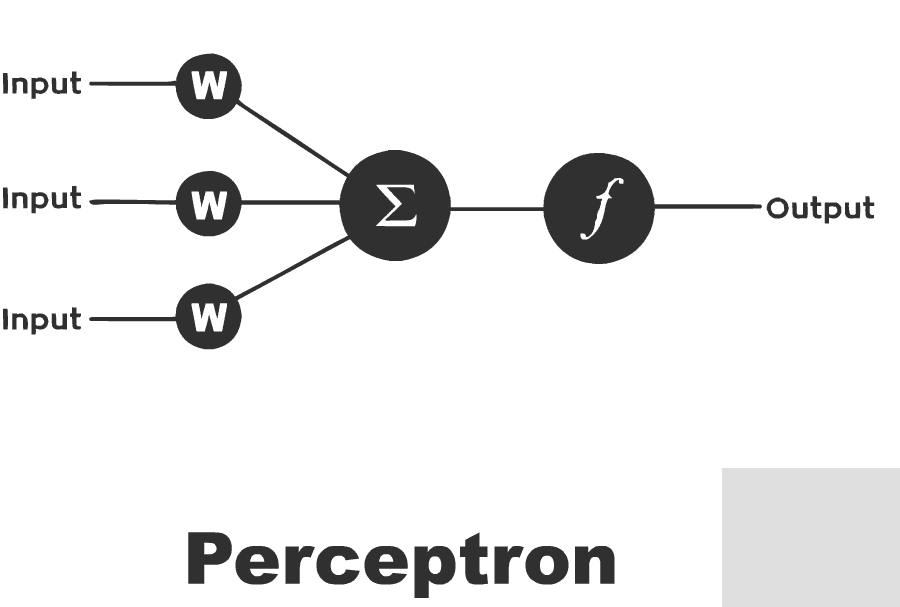The Perceptron is an algorithm for supervised learning of binary classifiers. functions that can decide whether an input (represented by a vector of numbers) belongs to one class or another.

Much like logistic regression, the weights in a neural net are being multiplied by the input vertor summed up and feeded into the activation function's input.

A Perceptron Network can be designed to have multiple layers, leading to the Multi-Layer Perceptron (aka MLP)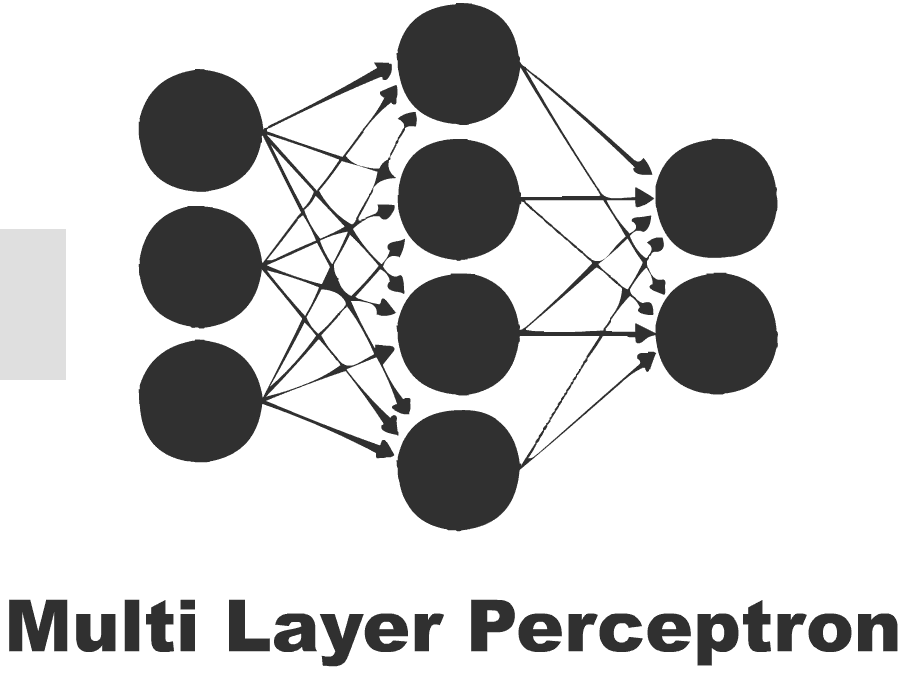# Single Layer Neural Network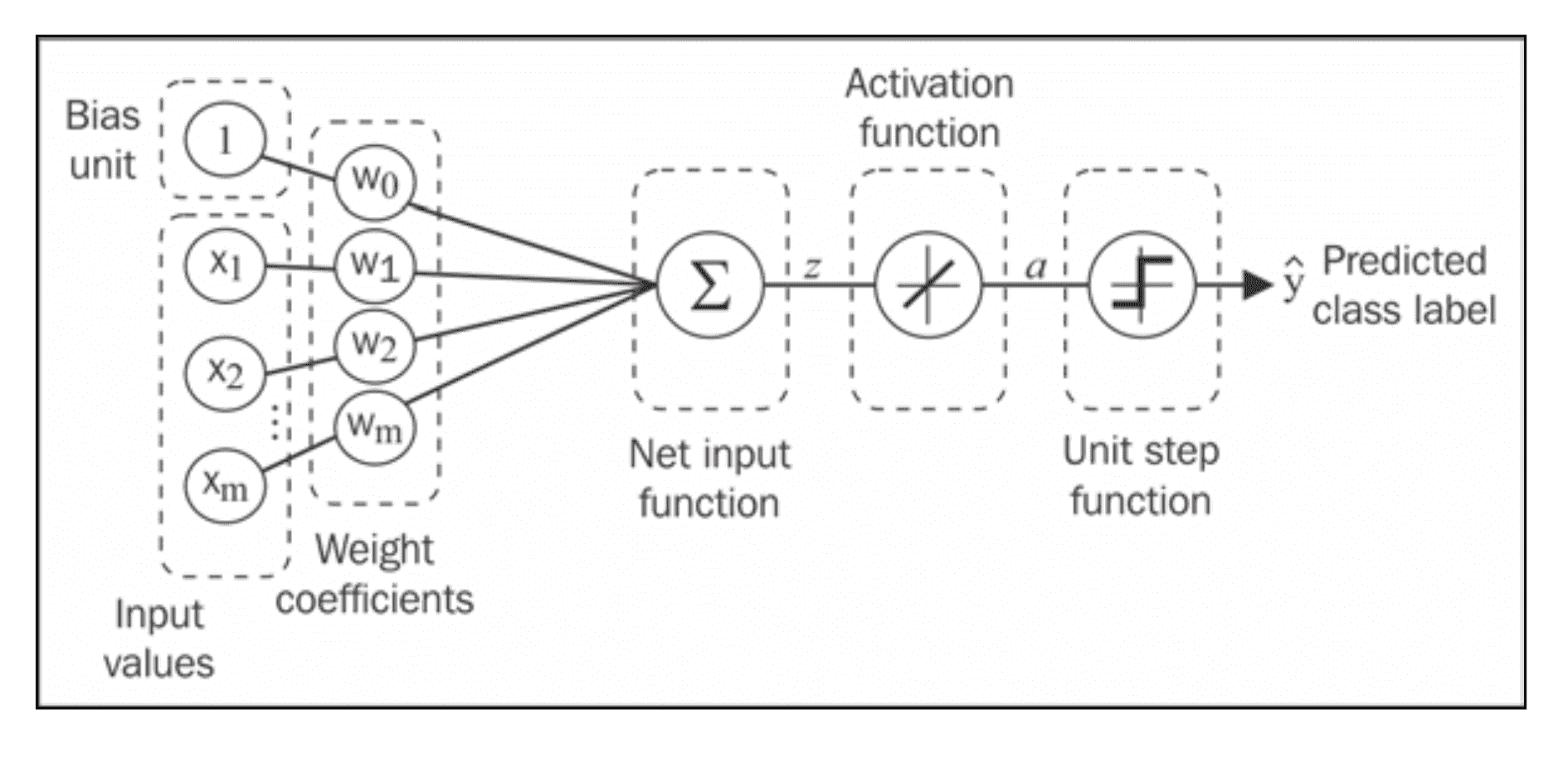(Source: Python Machine Learning, S. Raschka)

### Weights Update Rule

• We use a gradient descent optimization algorithm to learn the Weights Coefficients of the model.

• In every epoch (pass over the training set), we update the weight vector $w$ using the following update rule:

$$w = w + \Delta w, \text{where } \Delta w = - \eta \nabla J(w)$$

In other words, we computed the gradient based on the whole training set and updated the weights of the model by taking a step into the opposite direction of the gradient $\nabla J(w)$.

In order to fin the optimal weights of the model, we optimized an objective function (e.g. the Sum of Squared Errors (SSE)) cost function $J(w)$.

Furthermore, we multiply the gradient by a factor, the learning rate $\eta$ , which we choose carefully to balance the speed of learning against the risk of overshooting the global minimum of the cost function.

In gradient descent optimization, we update all the weights simultaneously after each epoch, and we define the partial derivative for each weight $w_j$ in the weight vector $w$ as follows:

$$\frac{\partial}{\partial wj} J(w) = \sum{i} ( y^{(i)} - a^{(i)} ) x^{(i)}_j$$

Note: The superscript $(i)$ refers to the i-th sample. The subscript $j$ refers to the j-th dimension/feature

Here $y^{(i)}$ is the target class label of a particular sample $x^{(i)}$ , and $a^{(i)}$ is the activation of the neuron

(which is a linear function in the special case of Perceptron).

We define the activation function $\phi(\cdot)$ as follows:

$$\phi(z) = z = a = \sum_{j} w_j x_j = \mathbf{w}^T \mathbf{x}$$

### Binary Classification

While we used the activation $\phi(z)$ to compute the gradient update, we may use a threshold function (Heaviside function) to squash the continuous-valued output into binary class labels for prediction:

$$\hat{y} = \begin{cases} 1 & \text{if } \phi(z) \geq 0 \ 0 & \text{otherwise} \end{cases}$$

## Building Neural Nets from scratch

### Idea:

We will build the neural networks from first principles. We will create a very simple model and understand how it works. We will also be implementing backpropagation algorithm.

Please note that this code is not optimized and not to be used in production.

This is for instructive purpose - for us to understand how ANN works.

Libraries like theano have highly optimized code.

Take a look at this notebook : Perceptron and Adaline

If you want a sneak peek of alternate (production ready) implementation of Perceptron for instance try:

from sklearn.linear_model import Perceptron


## Introducing the multi-layer neural network architecture(Source: Python Machine Learning, S. Raschka)

Now we will see how to connect multiple single neurons to a multi-layer feedforward neural network; this special type of network is also called a multi-layer perceptron (MLP).

The figure shows the concept of an MLP consisting of three layers: one input layer, one hidden layer, and one output layer.

The units in the hidden layer are fully connected to the input layer, and the output layer is fully connected to the hidden layer, respectively.

If such a network has more than one hidden layer, we also call it a deep artificial neural network.

### Notation

we denote the ith activation unit in the lth layer as $ai^{(l)}$ , and the activation units $a_0^{(1)}$ and $a_0^{(2)}$ are the bias units, respectively, which we set equal to $1$.

The _activation
of the units in the input layer is just its input plus the bias unit:

$$\mathbf{a}^{(1)} = [a_0^{(1)}, a_1^{(1)}, \ldots, a_m^{(1)}]^T = [1, x_1^{(i)}, \ldots, x_m^{(i)}]^T$$

Note: $x_j^{(i)}$ refers to the jth feature/dimension of the ith sample

### Notes on Notation (usually) Adopted

The terminology around the indices (subscripts and superscripts) may look a little bit confusing at first.

You may wonder why we wrote $w{j,k}^{(l)}$ and not $w{k,j}^{(l)}$ to refer to the weight coefficient that connects the kth unit in layer $l$ to the jth unit in layer $l+1$.

What may seem a little bit quirky at first will make much more sense later when we vectorize the neural network representation.

For example, we will summarize the weights that connect the input and hidden layer by a matrix

$$W^{(1)} \in \mathbb{R}^{h×[m+1]}$$

where $h$ is the number of hidden units and $m + 1$ is the number of hidden units plus bias unit.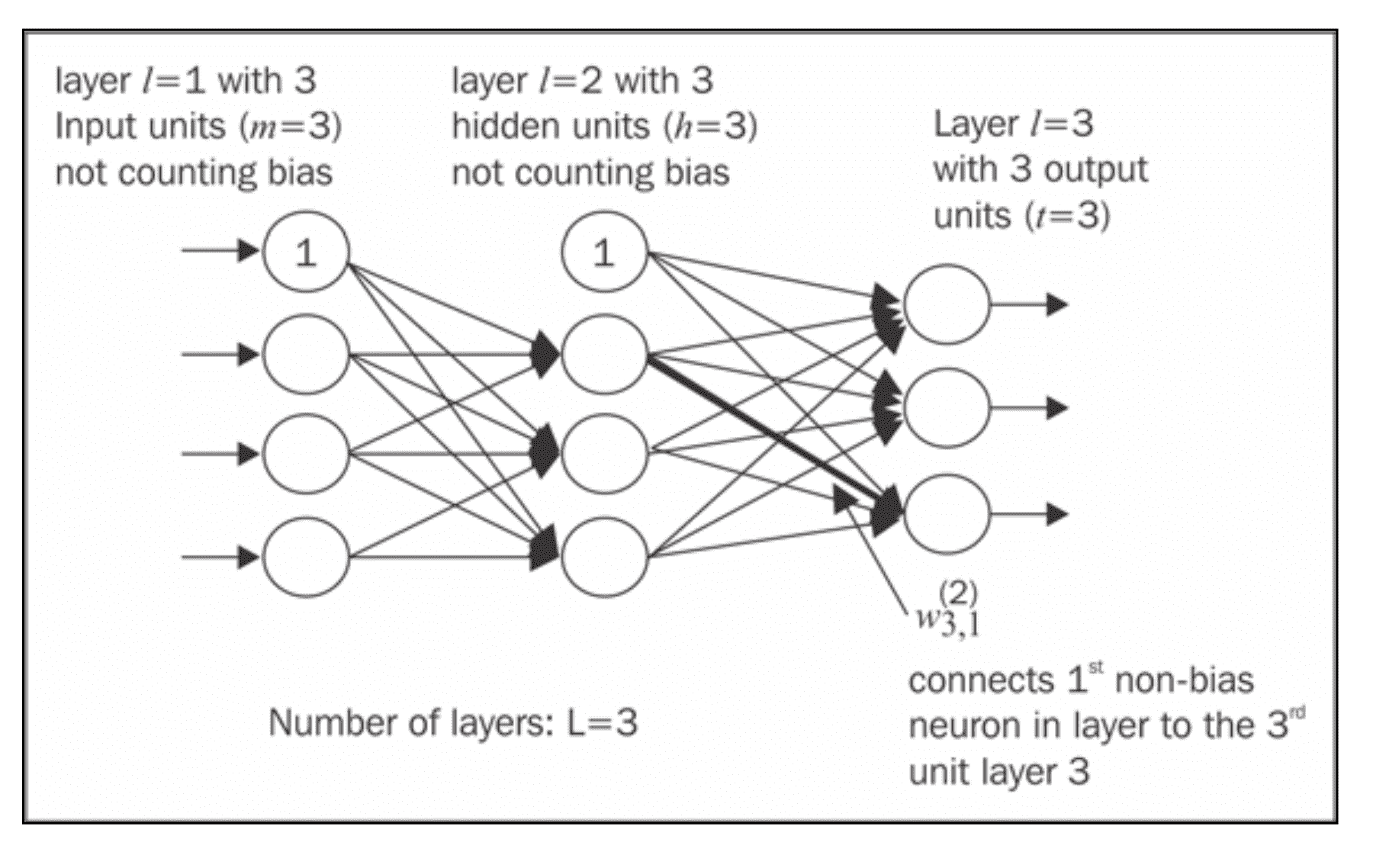(Source: Python Machine Learning, S. Raschka)

## Forward Propagation

• Starting at the input layer, we forward propagate the patterns of the training data through the network to generate an output.

• Based on the network's output, we calculate the error that we want to minimize using a cost function that we will describe later.

• We backpropagate the error, find its derivative with respect to each weight in the network, and update the model.

### Sigmoid Activation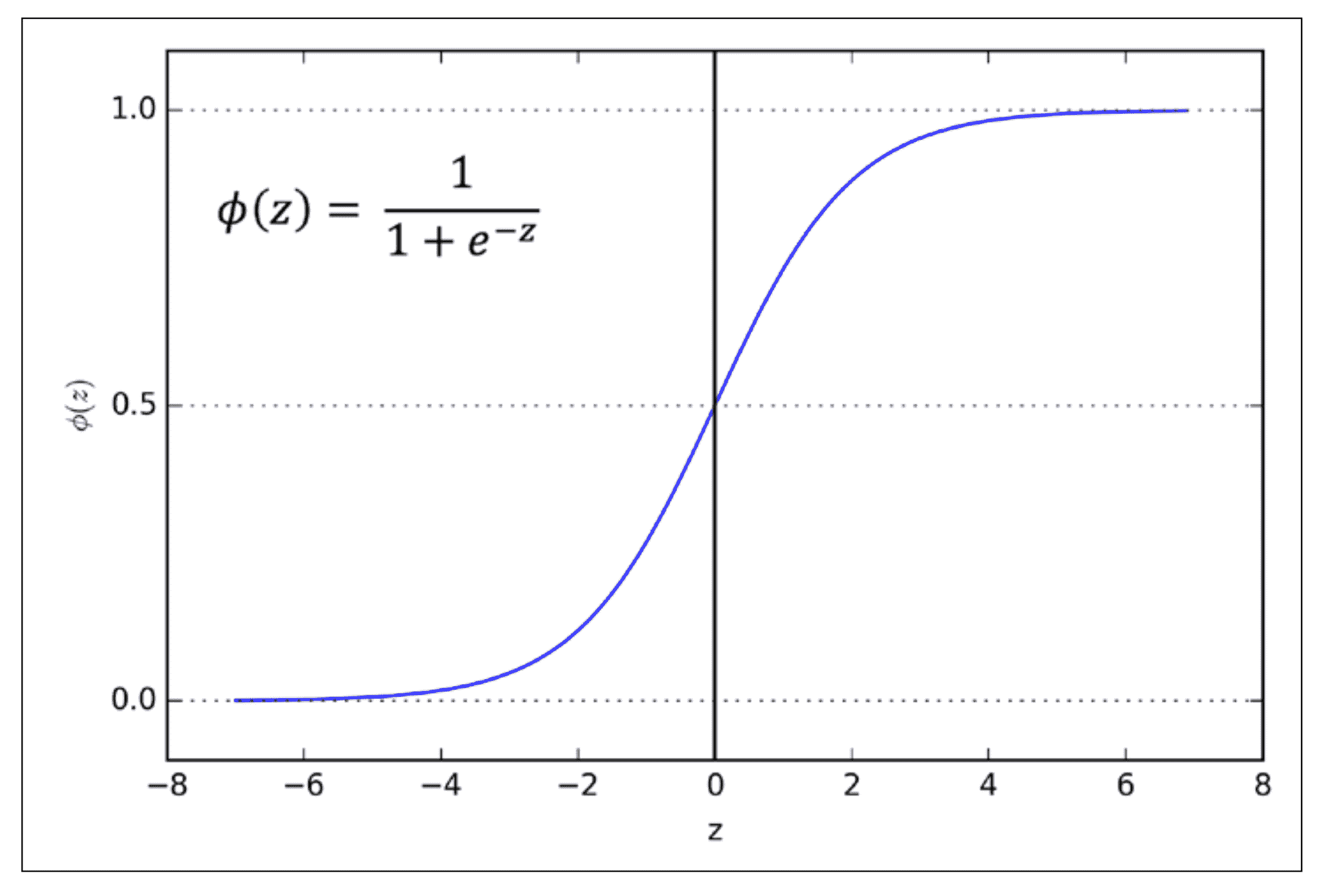(Source: Python Machine Learning, S. Raschka)(Source: Python Machine Learning, S. Raschka)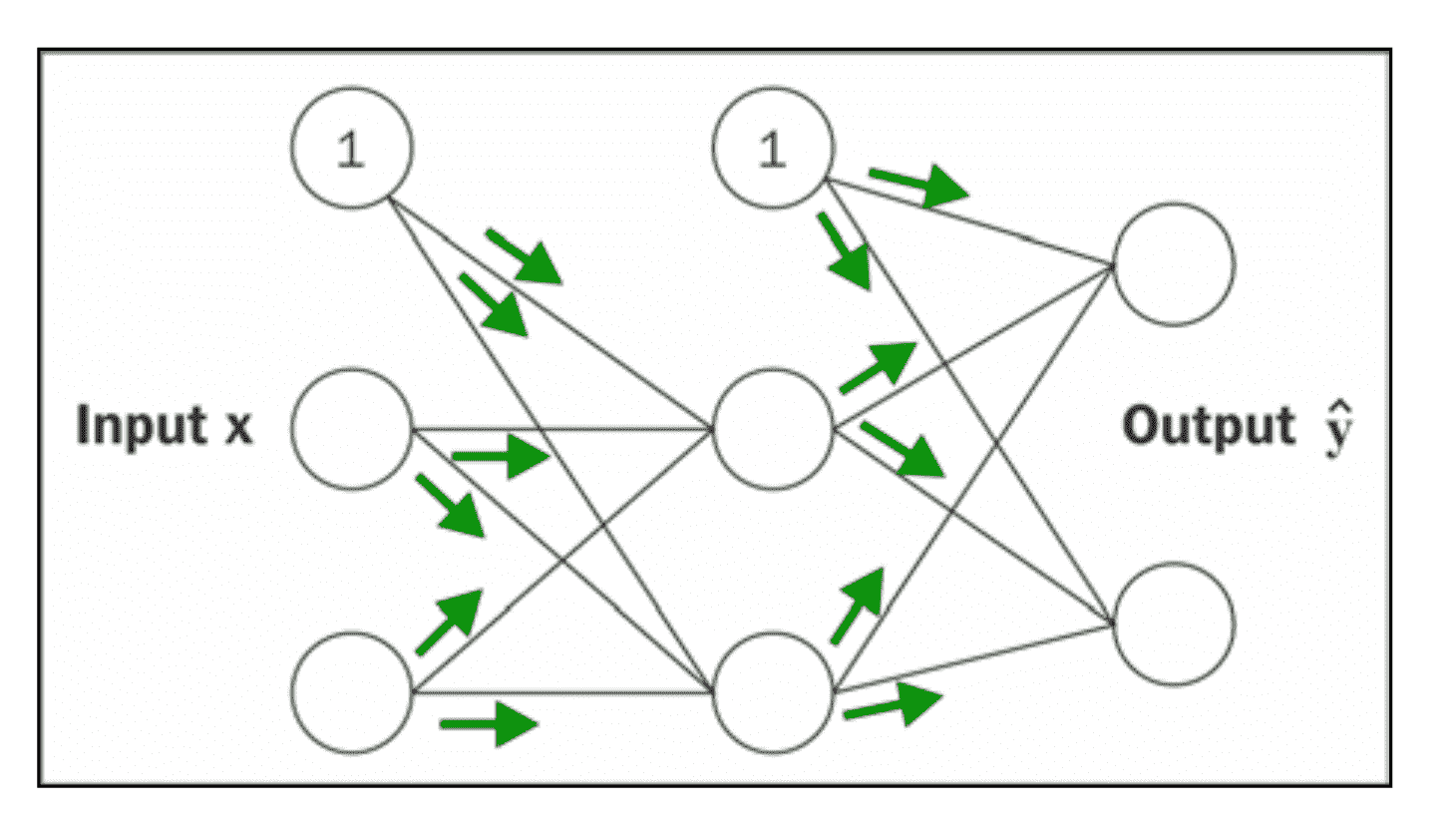(Source: Python Machine Learning, S. Raschka)

## Backward Propagation

The weights of each neuron are learned by gradient descent, where each neuron's error is derived with respect to it's weight.(Source: Python Machine Learning, S. Raschka)

Optimization is done for each layer with respect to the previous layer in a technique known as BackPropagation.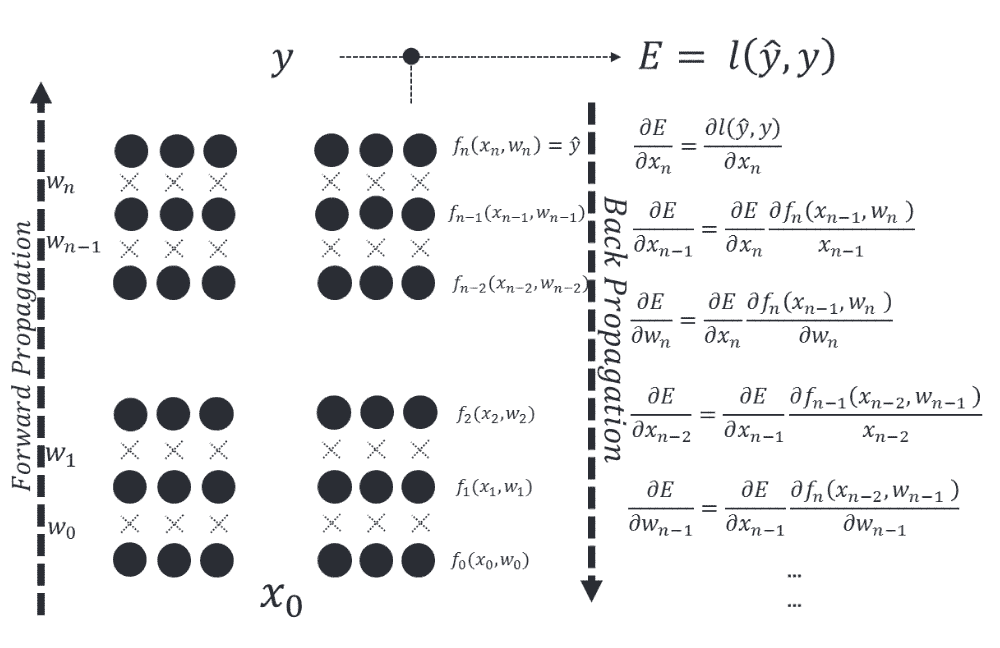(The following code is inspired from these terrific notebooks)

# Import the required packages
import numpy as np
import pandas as pd
import matplotlib
import matplotlib.pyplot as plt
import scipy

# Display plots in notebook
%matplotlib inline
# Define plot's default figure size
matplotlib.rcParams['figure.figsize'] = (10.0, 8.0)

#read the datasets

X, y = np.array(train.ix[:,0:2]), np.array(train.ix[:,2])

X.shape

(500, 2)

y.shape

(500,)

#Let's plot the dataset and see how it is
plt.scatter(X[:,0], X[:,1], s=40, c=y, cmap=plt.cm.BuGn)

<matplotlib.collections.PathCollection at 0x10e9329b0>## Start Building our MLP building blocks

Note: This process will eventually result in our own Neural Networks class

### A look at the details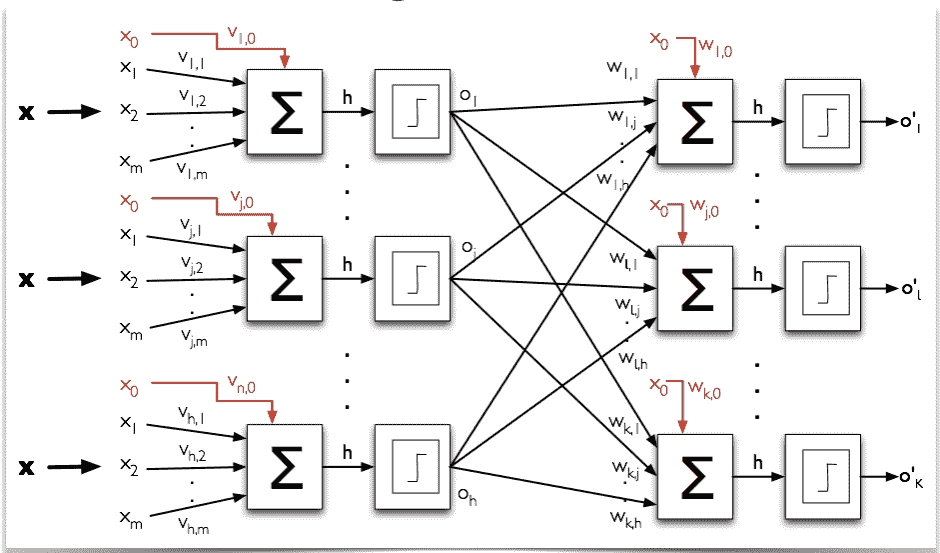import random
random.seed(123)

# calculate a random number where:  a <= rand < b
def rand(a, b):
return (b-a)*random.random() + a

##### Function to generate a random number, given two numbers

Where will it be used?: When we initialize the neural networks, the weights have to be randomly assigned.

# Make a matrix
def makeMatrix(I, J, fill=0.0):
return np.zeros([I,J])


### Define our activation function. Let's use sigmoid function

# our sigmoid function
def sigmoid(x):
#return math.tanh(x)
return 1/(1+np.exp(-x))


### Derivative of our activation function.

Note: We need this when we run the backpropagation algorithm

# derivative of our sigmoid function, in terms of the output (i.e. y)
def dsigmoid(y):
return y - y**2


### Our neural networks class

When we first create a neural networks architecture, we need to know the number of inputs, number of hidden layers and number of outputs.

The weights have to be randomly initialized.

class MLP:
def __init__(self, ni, nh, no):
# number of input, hidden, and output nodes
self.ni = ni + 1 # +1 for bias node
self.nh = nh
self.no = no

# activations for nodes
self.ai = [1.0]*self.ni
self.ah = [1.0]*self.nh
self.ao = [1.0]*self.no

# create weights
self.wi = makeMatrix(self.ni, self.nh)
self.wo = makeMatrix(self.nh, self.no)

# set them to random vaules
self.wi = rand(-0.2, 0.2, size=self.wi.shape)
self.wo = rand(-2.0, 2.0, size=self.wo.shape)

# last change in weights for momentum
self.ci = makeMatrix(self.ni, self.nh)
self.co = makeMatrix(self.nh, self.no)


### Activation Function

def activate(self, inputs):

if len(inputs) != self.ni-1:
print(inputs)
raise ValueError('wrong number of inputs')

# input activations
for i in range(self.ni-1):
self.ai[i] = inputs[i]

# hidden activations
for j in range(self.nh):
sum_h = 0.0
for i in range(self.ni):
sum_h += self.ai[i] * self.wi[i][j]
self.ah[j] = sigmoid(sum_h)

# output activations
for k in range(self.no):
sum_o = 0.0
for j in range(self.nh):
sum_o += self.ah[j] * self.wo[j][k]
self.ao[k] = sigmoid(sum_o)

return self.ao[:]


### BackPropagation

def backPropagate(self, targets, N, M):

if len(targets) != self.no:
print(targets)
raise ValueError('wrong number of target values')

# calculate error terms for output
output_deltas = np.zeros(self.no)
for k in range(self.no):
error = targets[k]-self.ao[k]
output_deltas[k] = dsigmoid(self.ao[k]) * error

# calculate error terms for hidden
hidden_deltas = np.zeros(self.nh)
for j in range(self.nh):
error = 0.0
for k in range(self.no):
error += output_deltas[k]*self.wo[j][k]
hidden_deltas[j] = dsigmoid(self.ah[j]) * error

# update output weights
for j in range(self.nh):
for k in range(self.no):
change = output_deltas[k] * self.ah[j]
self.wo[j][k] += N*change +
M*self.co[j][k]
self.co[j][k] = change

# update input weights
for i in range(self.ni):
for j in range(self.nh):
change = hidden_deltas[j]*self.ai[i]
self.wi[i][j] += N*change +
M*self.ci[i][j]
self.ci[i][j] = change

# calculate error
error = 0.0
for k in range(len(targets)):
error += 0.5*(targets[k]-self.ao[k])**2
return error

# Putting all together

class MLP:
def __init__(self, ni, nh, no):
# number of input, hidden, and output nodes
self.ni = ni + 1 # +1 for bias node
self.nh = nh
self.no = no

# activations for nodes
self.ai = [1.0]*self.ni
self.ah = [1.0]*self.nh
self.ao = [1.0]*self.no

# create weights
self.wi = makeMatrix(self.ni, self.nh)
self.wo = makeMatrix(self.nh, self.no)

# set them to random vaules
for i in range(self.ni):
for j in range(self.nh):
self.wi[i][j] = rand(-0.2, 0.2)
for j in range(self.nh):
for k in range(self.no):
self.wo[j][k] = rand(-2.0, 2.0)

# last change in weights for momentum
self.ci = makeMatrix(self.ni, self.nh)
self.co = makeMatrix(self.nh, self.no)

def backPropagate(self, targets, N, M):

if len(targets) != self.no:
print(targets)
raise ValueError('wrong number of target values')

# calculate error terms for output
output_deltas = np.zeros(self.no)
for k in range(self.no):
error = targets[k]-self.ao[k]
output_deltas[k] = dsigmoid(self.ao[k]) * error

# calculate error terms for hidden
hidden_deltas = np.zeros(self.nh)
for j in range(self.nh):
error = 0.0
for k in range(self.no):
error += output_deltas[k]*self.wo[j][k]
hidden_deltas[j] = dsigmoid(self.ah[j]) * error

# update output weights
for j in range(self.nh):
for k in range(self.no):
change = output_deltas[k] * self.ah[j]
self.wo[j][k] += N*change + M*self.co[j][k]
self.co[j][k] = change

# update input weights
for i in range(self.ni):
for j in range(self.nh):
change = hidden_deltas[j]*self.ai[i]
self.wi[i][j] += N*change + M*self.ci[i][j]
self.ci[i][j] = change

# calculate error
error = 0.0
for k in range(len(targets)):
error += 0.5*(targets[k]-self.ao[k])**2
return error

def test(self, patterns):
self.predict = np.empty([len(patterns), self.no])
for i, p in enumerate(patterns):
self.predict[i] = self.activate(p)
#self.predict[i] = self.activate(p)

def activate(self, inputs):

if len(inputs) != self.ni-1:
print(inputs)
raise ValueError('wrong number of inputs')

# input activations
for i in range(self.ni-1):
self.ai[i] = inputs[i]

# hidden activations
for j in range(self.nh):
sum_h = 0.0
for i in range(self.ni):
sum_h += self.ai[i] * self.wi[i][j]
self.ah[j] = sigmoid(sum_h)

# output activations
for k in range(self.no):
sum_o = 0.0
for j in range(self.nh):
sum_o += self.ah[j] * self.wo[j][k]
self.ao[k] = sigmoid(sum_o)

return self.ao[:]

def train(self, patterns, iterations=1000, N=0.5, M=0.1):
# N: learning rate
# M: momentum factor
patterns = list(patterns)
for i in range(iterations):
error = 0.0
for p in patterns:
inputs = p
targets = p
self.activate(inputs)
error += self.backPropagate([targets], N, M)
if i % 5 == 0:
print('error in interation %d : %-.5f' % (i,error))
print('Final training error: %-.5f' % error)


### Running the model on our dataset

# create a network with two inputs, one hidden, and one output nodes
ann = MLP(2, 1, 1)

%timeit -n 1 -r 1 ann.train(zip(X,y), iterations=2)

error in interation 0 : 53.62995
Final training error: 53.62995
Final training error: 47.35136
1 loop, best of 1: 36.7 ms per loop


### Predicting on training dataset and measuring in-sample accuracy

%timeit -n 1 -r 1 ann.test(X)

1 loop, best of 1: 11.8 ms per loop

prediction = pd.DataFrame(data=np.array([y, np.ravel(ann.predict)]).T,
columns=["actual", "prediction"])

actual prediction
0 1.0 0.491100
1 1.0 0.495469
2 0.0 0.097362
3 0.0 0.400006
4 1.0 0.489664
np.min(prediction.prediction)

0.076553078113180129


### Let's visualize and observe the results

# Helper function to plot a decision boundary.
# This generates the contour plot to show the decision boundary visually
def plot_decision_boundary(nn_model):
# Set min and max values and give it some padding
x_min, x_max = X[:, 0].min() - .5, X[:, 0].max() + .5
y_min, y_max = X[:, 1].min() - .5, X[:, 1].max() + .5
h = 0.01
# Generate a grid of points with distance h between them
xx, yy = np.meshgrid(np.arange(x_min, x_max, h),
np.arange(y_min, y_max, h))
# Predict the function value for the whole gid
nn_model.test(np.c_[xx.ravel(), yy.ravel()])
Z = nn_model.predict
Z[Z>=0.5] = 1
Z[Z<0.5] = 0
Z = Z.reshape(xx.shape)
# Plot the contour and training examples
plt.contourf(xx, yy, Z, cmap=plt.cm.Spectral)
plt.scatter(X[:, 0], X[:, 1], s=40,  c=y, cmap=plt.cm.BuGn)

plot_decision_boundary(ann)
plt.title("Our initial model")

<matplotlib.text.Text at 0x110bafd68>Exercise:

Create Neural networks with 10 hidden nodes on the above code.

What's the impact on accuracy?

# Put your code here
#(or load the solution if you wanna cheat :-)

# %load ../solutions/sol_111.py


Exercise:

Train the neural networks by increasing the epochs.

What's the impact on accuracy?

#Put your code here

# %load ../solutions/sol_112.py